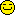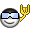Original Post
Flame Forge Basics
This is a list of what all the sliders do individually. There are a lot of different things doable from combining certain effects, but my list of currently interesting effects of combinations is fairly long. Such advanced techniques make each individual forger unique, so thus I will not divulge them for the sake of preserving variation in creativity, style, and profitable trade secrets.

Require 3.72 or later. To get to flame forge, press ctrl+L ingame.

THIS IS NOT A PLACE TO ASK HOW CERTAIN EFFECTS ARE MADE. This is just a tutorial list of what the slider functions are. Questions to clarify what a function does is acceptable.

bodypart: see this for body parts
The flame particles are generated on this bodypart number.

timestep: overall speed. more is faster

damping: inverted value for deceleration/"air drag". 1 = none. 0 = max.

blend: 1 = additive color, else negative color. On 1, colors add on. A (255,0,0) particle on a (0,255,0) background makes yellow (255,255,0). Thus, it's "invisible" on a white background. Else, particle color is subtracted. Background of (255,255,255) minus particle (255,0,0) is cyan (0,255,255).

sink: 0 means particles don't get absorbed into the ground, while 1 means they do. While it may seem fun to turn it off, the fake reflection when the option is on makes it look odd.

color_r,g,b,a,random: Red, Green, Blue starting particle colors, effect depends on blend. Alpha only matters for blend=1. random gives random start colors and disables other RGB values.

target_color_r,g,b,a,random: RGBA that all particles will change to from start color at a rate depending on target_color_scale.

target_color_scale: rate at which particles change color towards target_r,g,b,a,random.

age_start,limit: the difference determines the lifetime of the particle.

age_sigma: indeterminable. supposedly variation and probability range of lifetimes.

emit_scale: size of emission source, from 1 (the size of the bodypart) to 2 (double width/height/depth)

emit_amount: rate of particles emitted, varies naturally

size: starting size at emission source

size_random: random amount to randomly add/subtract. size is maxed at 100.

target_size: ending size

target_size_scale: rate at which size changes towards target_size

target_size_random: random amount to randomly add/subtract to target_size

random_displace_x,y,z: amount to randomly add/subtract to particle positions in given direction. Gives jitter.

random_displace_random: applies to all three directions.

rotatable: 1 makes velocity_x,y,z directions relative to bodypart rotation

gravity_x,y,z: acceleration in each direction.

velocity_x,y,z: starting velocity of particles from emission source

velocity_random: random starting velocity

ORBIT: enables orbit. Provides an acceleration towards a bodypart inversely proportional to radial distance, much like gravity.

orbit_bodypart: target of orbit

orbit_magnitude: multiplier of acceleration given to particles in direction of orbit_bodypart

orbit_epsilon: orbit's effect dampener at close distances

GRAVITATE: enables gravitate. Adds acceleration towards a mass of particles "center of mass"

gravitate_magnitude: multiplier on acceleration given.

gravitate_epsilon: gravitate's effect dampener

FOLLOW: enables follow. Adds acceleration to particles from every particle towards every other particle.

follow_magnitude: multiplier on acceleration given.

follow_epsilon: follow's effect dampener

SINK_BODY: enables sink_body. This removes particles from an area around a bodypart

sink_body_bodypart: bodypart to remove particles near to it

sink_body_radius: distance from bodypart which sink_body will remove particles
Last edited by WorldEater; Nov 2, 2017 at 08:54 PM.
nice tut.

+ rep

didn't know the epsilon thing. Also, mind explainging how follow accelerates particles?
- its been a while
^
follow makes particles constantly accelerate towards the point, different from gravity because gravity uses the inverse of the distance.

Very useful info mateYour post could do with some formatting though, perhaps bold the flame field name >.>
When I see you, my heart goes DOKI⑨DOKI
Fish: "Gorman has been chosen for admin. After a lengthy discussion we've all decided that Gorman is the best choice for the next admin."
Where do you press Ctrl+shift+alt+I in?? To open it
T.dot
Thanks for the tut... it's very helpfullDon't mess with me ;)
Very Proud member of OLDA!
10th Dan Black Belt
Join Date: Jul 2008
Posts: 3,416
Where do you press Ctrl+shift+alt+I in?? To open it

You have to press ctrl+L ingame.
Junior Member
Blue Belt
Join Date: Jul 2009
Posts: 1
how much is flame forger, and i cant get one :O
10th Dan Black Belt
Join Date: Jul 2008
Posts: 3,416
http://forum.toribash.com/tori_boosters.php

25\$ per month

You need a paypal.
3rd Dan Black Belt
Join Date: Mar 2009
Posts: 2,960
Loving this tutorial, You should totally Update it with the new stuffs so i dont have to harass you every time I need help.
What new stuff? This is all that there is.
Theres a lot of tricks that involve using more than one setting, but I can't make a comprehensive tut for that.